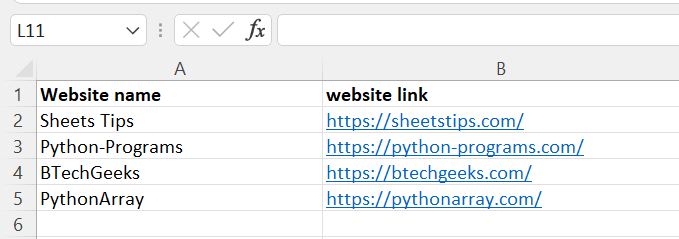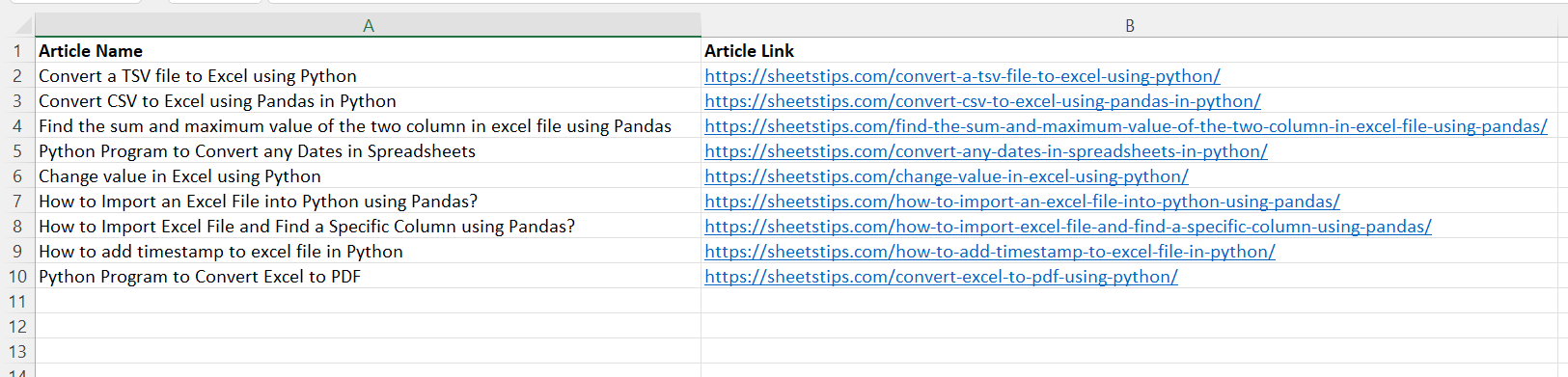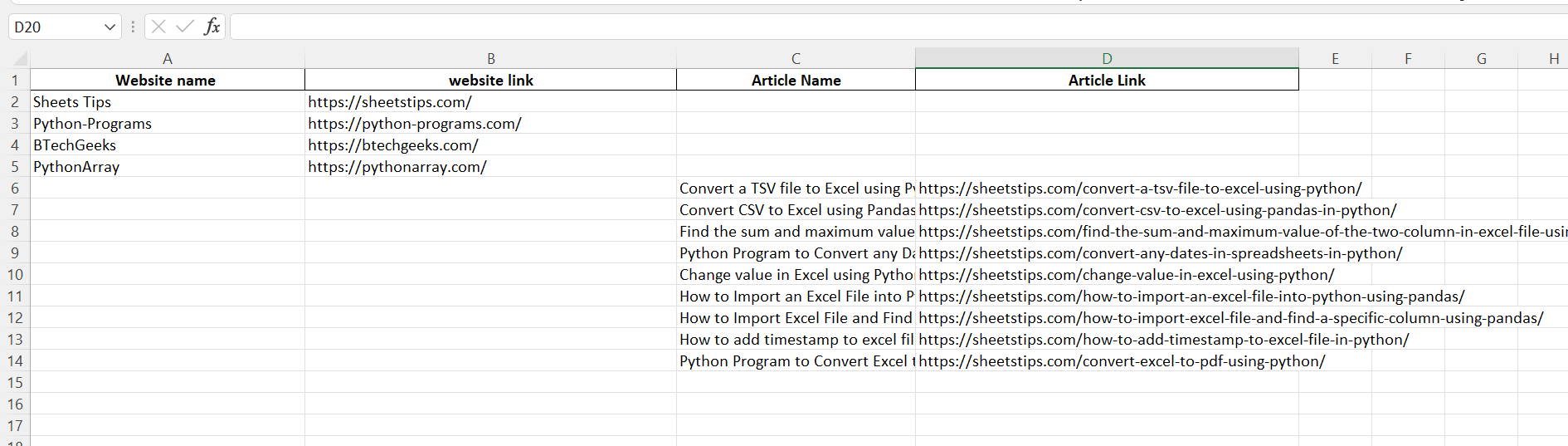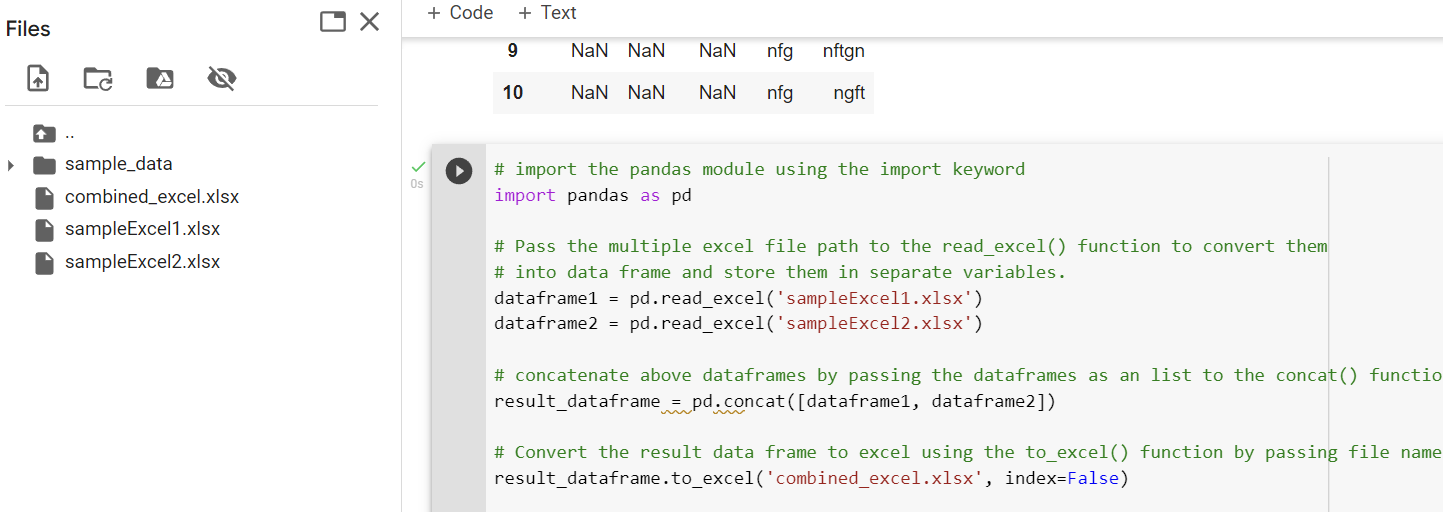# Combine Multiple Excel Worksheets Into a Single Pandas Dataframe

Let us assume that you have two Excel files of the same structure, for example, Excel1.xlsx and Excel2.xlsx. Now we want to merge both of these sheets into a new Excel file. To get the same done, we can simply use Pandas. Yes, you heard it right. We can convert and combine multiple excel worksheets into a single file using Pandas Dataframe. Let us discuss the detailed steps for converting multiple Excel files into a single Pandas data frame on this page. Read further to find out more.

## Prerequisites For Combining Multiple Excel Worksheets into a Single Pandas Dataframe

The prerequisites to combine multiple excel worksheets/files of the same structure into a single sheet are given below.

## Combine Multiple Excel Worksheets Into a Single Pandas Dataframe

Excel sheet 1(SampleExcel1.xlsx):Excel sheet 2(SampleExcel2.xlsx):to_excel() function:

To export the DataFrame to an excel file, use the to_excel() method. The target file name must be specified when writing a single object to an excel file. If we wish to write to many sheets, we must first construct an ExcelWriter object with the target filename and then indicate the sheet in the file we want to write to. The unique sheet name can also be used to write numerous sheets. All modifications made to the data written to the file must be saved.

The read excel method accepts two arguments: sheet name and index col. sheet name defines the sheet from which the data frame should be created, and the index col gives the title column.

Approach:

• Import the pandas module using the import keyword
• Pass the multiple excel file path to the read_excel() function to convert them into data frame and store them in separate variables.
• Concatenate the above dataframes by passing the dataframes as a list to the concat() function of the pandas module.
• Convert the result data frame to excel using the to_excel() function by passing the file name as argument and index as per user choice(True/False).
• The Exit of the Program

Below is the Implementation:

```# import the pandas module using the import keyword
import pandas as pd

# Pass the multiple excel file path to the read_excel() function to convert them
# into data frame and store them in separate variables.

# Concatenate the above dataframes by passing the dataframes as a list to the concat() function of the pandas module.
result_dataframe = pd.concat([dataframe1, dataframe2])

# Convert the result data frame to excel using the to_excel()
# function by passing the file name as argument and index as per user choice(True/False).
result_dataframe.to_excel('combined_excel.xlsx', index=False)
```

Output Image Sample: